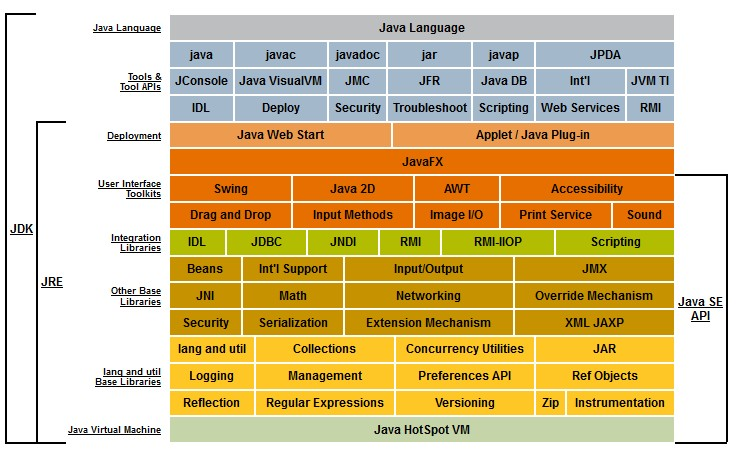# Java - long - Long (integer 64 bit)

In Java, the long data type stores integer on 64 bit while the integer data type stores integer on 32bit.

The primitive wrapper Long is a subclass of Number in java

## Implementation

### Primitive Integer

Long is an integer that is encoded with 64 bit but you have also int on 32 bit.

Primitive Type Primitive Wrapper Stored in a word of min max
int Integer 32 bits $-2^{31}$
-2,147,483,648
$+2^{31}-1$
-2,147,483,647
long Long 64 bits $-2^{63}$
-9,223,372,036,854,775,807
$+2^{63-1}$
‭4.611.686.018.427.387.904‬

## Management

### Initialization

long = 1.073.741.824L;

• From a variable
long = (long)a;

• from an integer
Long i = theinteger.longValue()

• from an int
Long i = Long.valueOf(3);


### Operation

The operations are done in the largest data type required to handle all of the current values.

For the below mathematics Operations The operation is a
int (operator) int integer operation
int (operator) long long operation

Example:

• a long operation
long i = (int)1 * (long)2

• a int operation
long i = (int)1 * (int)2


### To Int

Long theLong;
Integer i = theLong.intValue()

• or from a long primitive
Math.toIntExact(long)


### To Array

new Long[]{long1, long2, ...};


Discover MoreJava - Integer

integer data type in Java. int (or Integer) is the 32 bit implementation of an integer. Java can also store an integer on 64 bit with a long. An integer in java is a sub-class of number They are...